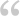Populating Vectors When They’re Created

Ever wonder how to populate a Vector when you create it?

Here’s a way: Instead of using the *new* operator to create the Vector use global conversion to convert an Array into a Vector (note that the *new* operator is not used in the sample):

 1 var samples : Vector. = Vector.( [ 1.0, 2.0, 3.0 ] );

Another pretty straightforward technique is to instantiate an empty Vector then push all the values with a single push method call:

 1 2 var samples2 : Vector. = new Vector.(); samples2.push( 4.0, 5.0, 6.0 );

You may wonder if you can pass an Array to a Vector constructor — nope, the Vector signature specifies 2 parameters: length of the Vector and a Boolean that can be used for locking the length of the Vector. Passing an Array to a Vector constructor yields an empty Vector.

 1 2 3 // Sample that fails to create a pre-populated Vector, // but it does create an empty Vector. var samples3 : Vector. = new Vector.( [ 7.0, 8.0, 9.0 ] );
 1 2 3 4 // Output from prior 3 samples trace( "samples: " + samples ); // output: samples: 1,2,3 trace( "samples2: " + samples2 ); // output: samples2: 4,5,6 trace( "samples3: " + samples3 ); // output: smaples3 (an empty Vector was created)

One ResponseLeave one →

1.DaveW

/  March 20, 2012Another way, slightly less typing than the first example:

 1 var samples4 : Vector. = new [3, 4.2, 6.83];

That syntax doesn’t work in Flash CS4, but it works in CS5 and above. I think it also didn’t work pre Flex SDK 4.0.